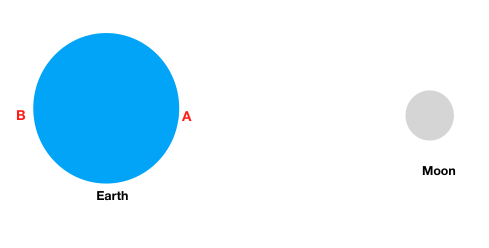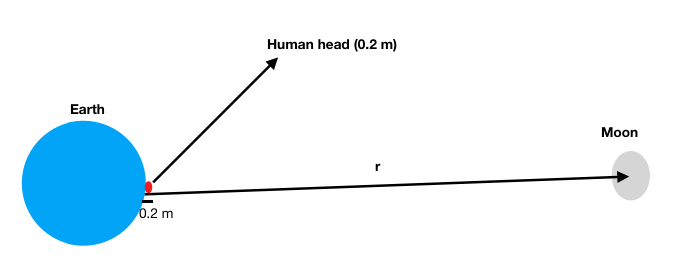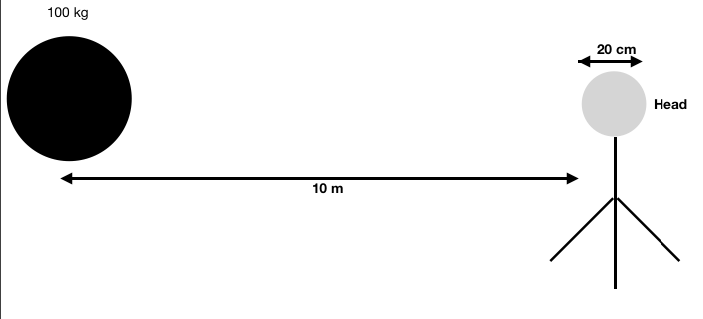## Pages

### Using gravitational law to verify proclaimed effects of moon’s gravitation on humans’ mental health during full moon day

Lunar eclipse is an eventful day on earth because of various beliefs attached with it. Two popular beliefs are that food gets poisoned and the mental health of humans is affected, with pronounced effects in people with previous history of mental health issues. Though this is seen specifically on lunar eclipse, people also claim that similar effects on mental health front also happen on full moon day.

Supporters of the claim that moon affects human mental health on lunar eclipse or full moon day, claim that, such an effect is due to the gravitation force of moon on human head, that creates micro tides in our head, in the way moon creates tides on earth. This post cross checks this claims using Newton’s formula for gravitational force between two bodies. The structure of the post is as follows
1. Background: Simple explanation for cause of tides on earth due to Moon
2. Tidal gravitational force of Moon on human head
3. Tidal gravitational force of a 100 kg terrestrial object on human head
4. Conclusion: Comparing tidal gravity of moon and 100 kg object on human’s head.

### I. Background: Simple explanation for cause of tides on earth due to Moon

The primary mechanism of tides on earth is what we call “tidal gravity”. In simple words, tidal gravity is the difference in gravitational force on an object at its two ends.Gravitational force of Moon at point A is greater than gravitational force at point B. Image not to scale
As we can see from the picture above, gravitational force of moon at point A is greater than gravitational force at point B. Due to the difference in forces, the liquid on the outer surface of the earth gets stretched causing a bulge.
Contrary to what we think of tides as “going out and coming in”, we are the ones going towards the bulge and moving away from the bulge, as the earth is rotating within this liquid outer surface. We perceive this movement of coming close to the bulge and moving away from the bulge, as the raise and fall of the tide.

Note that Sun also causes tidal forces on earth. When the tidal forces of Sun and Moon align together, we get the tides that we observe on a full moon day.

### II. Tidal gravitational force of Moon on a human head

Remember that the claim of lunar eclipse or full moon causing mental issues is based on the fact that moon causes similar effects on liquid in human head, the way it does on water on earth. So, it must mean that the tidal bulge created in our head due to moon’s force on liquid in our head causes the proclaimed harmful effect.

Let’s calculate the tidal force of moon on a human head. We all know that human head is not liquid. For simplicity sake, let’s assume that all of it is made up of liquid. Let’s calculate the tidal force of moon on human head, due to difference in moon’s gravitational force at the opposite ends of the head.

Newton’s formula for gravitational force between two objects of masses M and m, at a distance r says that such force

F = G*M*m/r²Tidal gravity of moon a human head. Distance of center of moon from surface of earth is r. Image not to scale
Tidal force of Moon on Human head (TF) = Gravity at point on human head closest to Moon — Gravity at point on human head farther from the moon

TF = [G*M*m/(r-0.2)²] — [G*M*m/r²]
TF (Moon on head) = [G*M*m][1/(r-0.2)²— 1/(r)²]
G = 6.67*10^-11 (N m²/kg²)
M = 7.35*10²² kg (mass of moon)
m = 5 kg (mass of head)
r = 356516600 (distance center of moon and center of earth — radius of earth = 356523000 m — 6400)
r — 0.2 = Distance between point on human head closest to the moon and the center of the moon
On putting these values in the formula for gravitational force, we get
TF (Moon on head) = 1.8* 10^-13 N

[You might have noted that we considered all the mass of head to be concentrated at closest point to moon, while calculating the gravitational force at that point. It’s not the case in reality as the mass is spread out; also the bulge at the end is a cumulative addition of stretch of liquid at points across the liquid surface. We are simplifying it for the sake of calculation and just measuring gravity at two end points, each time assuming that the whole mass is concentrated at these points when calculating it. We use a similar approximation in the next section also when calculating tidal force of 100 kg object on a human head.]

### III. Tidal gravitational force of 100 kg terrestrial object on a human head

Let’s consider a 100 kg object (a weightlifting dumbbell) whose center is at a distance of 10m from the closest end of the human head. As usual, head is of 5 kg and 20 cm in width/height. This is a common scenario we encounter in daily life.
Tidal force of a 100 kg earthly object on a head at a distance of 10m.Tidal gravity due to 100 kg object on human head at 10 m distance. Image not to sale
TF (Object on head) = [G*M*m][1/r² — 1/(r+0.2)²]
G = 6.67*10^-11 (N m²/kg²)
M = 100 kg
m = 5 kg
r = 10m
TF (Object on head) = 1.3*10^-11 N
The tidal force of a 100kg object on our head, when the object is at a distance of 10m from our head is 1.3*10^-11 N

### IV: Conclusion: Comparing tidal gravity of moon and 100 kg object on human’s head.

Compare the value of the tidal force of moon on human head (1.8 * 10^ -13 N) with the tidal force of 100 kg object on human head (1.3*10^-11 N)

We note that the tidal gravity of 100 kg earthly object on human head is 100 times greater than that of tidal force of Moon on human head.

If only micro-tides are caused in head on full moon day or a lunar eclipse day due to gravitational force of moon, resulting in mental issues, we should all be having mental issues all year long because many other terrestrial bodies have far higher tidal gravitational forces on human head, as compared to that of moon.

Further, if we make a 100 kg object placed at 10m from a human head, to oscillate vigorously, changing its distance from the human head, the resulting oscillation in gravitational force would turn us crazy — if this theory of wave creation inside head is the cause for mental illness.

Another fun calculation: Compare the tidal gravity of the pillow (on which you sleep) on the human’s head. You will be surprised by its magnitude as compared to the tidal gravity of moon on earth. Hint: Pillow’s tidal gravity on human head is far higher than that of tidal gravity of moon on human head. We should only wonder — why don’t we yet have pillow scare-mongers?